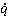### Create an Account

Home / Questions / Consider the clad fuel element of Problem 390 a Using appropriate relations from Tables C1...

# Consider the clad fuel element of Problem 390 a Using appropriate relations from Tables C1 and C2 obtain an expression for the temperature distribution Tx in the fuel element For

Consider the clad fuel element of Problem 3.90.

(a) Using appropriate relations from Tables C.1 and C.2, obtain an expression for the temperature distribution T(x) in the fuel element. For kf = 60 W/m ∙ K, L = 15 mm, b = 3 mm, ks = 15 W/m ∙ K, h = 10,000 W/m2 ∙ K, and T = 200°C, what are the largest and smallest temperatures in the fuel element if heat is generated uniformly at a volumetric rate of= 2 × 107 W/m3? What are the corresponding locations?

(b) If the insulation is removed and equivalent convection conditions are maintained at each surface, what is the corresponding form of the temperature distribution in the fuel element? For the conditions of part (a), what are the largest and smallest temperatures in the fuel? What are the corresponding locations?

(c) For the conditions of parts (a) and (b), plot the temperature distributions in the fuel element.

Jun 28 2020 View more View LessSubscribe To Get Solution